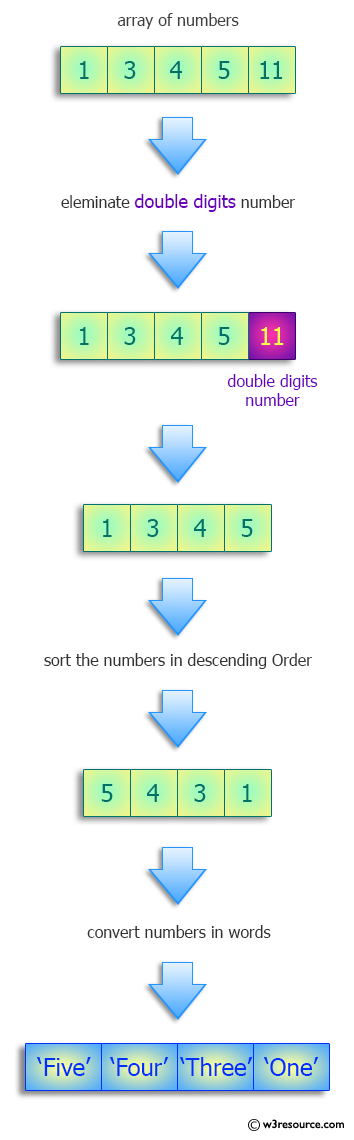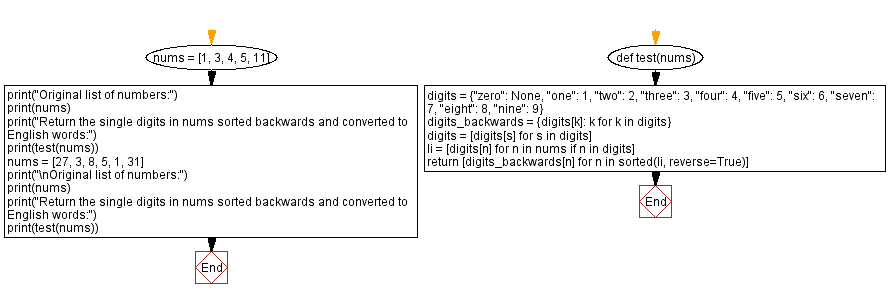﻿ Python: Single digits in numbers sorted backwards and converted to English words - w3resource# Python: Single digits in numbers sorted backwards and converted to English words

## Python Programming Puzzles: Exercise-96 with Solution

Write a Python program to get the single digits in numbers sorted backwards and converted to English words.

```Input:
[1, 3, 4, 5, 11]
Output:
['five', 'four', 'three', 'one']

Input:
[27, 3, 8, 5, 1, 31]
Output:
['eight', 'five', 'three', 'one']
```

Pictorial Presentation:Sample Solution:

Python Code:

``````#License: https://bit.ly/3oLErEI

def test(nums):
digits = {"zero": None,
"one": 1,
"two": 2,
"three": 3,
"four": 4,
"five": 5,
"six": 6,
"seven": 7,
"eight": 8,
"nine": 9}
digits_backwards = {digits[k]: k for k in digits}
digits = [digits[s] for s in digits]
li = [digits[n] for n in nums if n in digits]
return [digits_backwards[n] for n in sorted(li, reverse=True)]

nums = [1, 3, 4, 5, 11]
print("Original list of numbers:")
print(nums)
print("Return the single digits in nums sorted backwards and converted to English words:")
print(test(nums))
nums = [27, 3, 8, 5, 1, 31]
print("\nOriginal list of numbers:")
print(nums)
print("Return the single digits in nums sorted backwards and converted to English words:")
print(test(nums))
``````

Sample Output:

```Original list of numbers:
[1, 3, 4, 5, 11]
Return the single digits in nums sorted backwards and converted to English words:
['five', 'four', 'three', 'one']

Original list of numbers:
[27, 3, 8, 5, 1, 31]
Return the single digits in nums sorted backwards and converted to English words:
['eight', 'five', 'three', 'one']
```

Flowchart:## Visualize Python code execution:

The following tool visualize what the computer is doing step-by-step as it executes the said program:

Python Code Editor :

Have another way to solve this solution? Contribute your code (and comments) through Disqus.

What is the difficulty level of this exercise?

Test your Programming skills with w3resource's quiz.

﻿

## Python: Tips of the Day

Clamps num within the inclusive range specified by the boundary values x and y:

Example:

```def tips_clamp_num(num,x,y):
return max(min(num, max(x, y)), min(x, y))
print(tips_clamp_num(2, 4, 6))
print(tips_clamp_num(1, -1, -6))
```

Output:

```4
-1
```